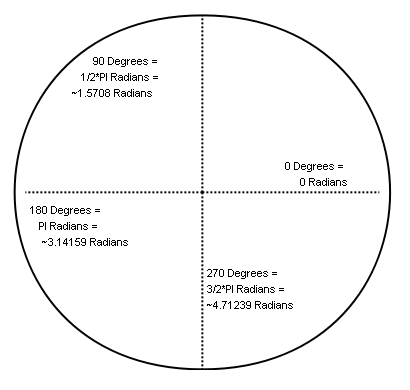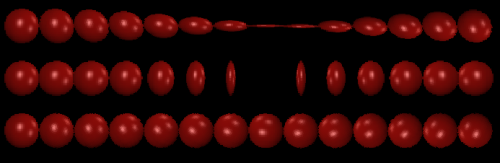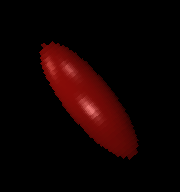# FlatRedBall.Math.IRotatable

## Introduction

Rotation controls the 3D orientation of PositionedObjects. Although some PositionedObjects such as Sprites and Text objects are 2D, all PositionedObjects can be rotated in 3D space.

Rotation can be represented using the RotationMatrix property or individual rotation components.

These components (RotationX, RotationY, RotationZ) are measured in radians, not degrees:For more information on working with rotations, see the Rotation wiki entry.

## The Math of Rotation

IRotatables represent their rotations as individual components (RotationX, RotationY, and Rotation) as well as a combined RotationMatrix which is of Microsoft.Xna.Framework.Matrix type.

For a conceptual understanding of matrices, see the Matrix wiki entry.

For more information, see our Trigonometry wiki entry to understand more about how rotations work.

### Degrees vs. Radians

If you recall your math classes, you may be familiar with the degree measurement. FlatRedBall uses radians as a measurement for its rotations. For more information on radians vs. degrees and why FlatRedBall uses radians, see the radians and degrees section on the Trigonometry page.

## IRotatable Properties

Rotation can be directly controlled in two different ways. Just like position and velocity, rotation exposes three individual properties which can be individually modified to rotate an object (RotationX, RotationY, RotationZ).

These are generally modified only when one axis is being changed on an object. Although it is possible to perform full 3D rotation by changing all three components individually, the order of rotation may cause unexpected results. When more complex rotation is required, use of the RotationMatrix property is recommended. This can be set to any matrix and the PositionedObject’s orientation will reflect the change. Also, the rotational components and RotationMatrix will reflect each other. Keep in mind that as the RotationMatrix is a property, it must be set and its individual values should not be changed. In other words, this is fine:

```someObject.RotationMatrix = someOtherMatrix;
```

But this is not:

```someObject.RotationMatrix.M11 = .135f;
```

## Using Rotation

The following code creates three rows of Sprites. Each row is rotated on a different axis.

``` for (int i = 0; i < 14; i++)
{
Sprite sprite = SpriteManager.AddSprite("redball.bmp");
sprite.X = -14 + i * 32;
sprite.Y = 3;
sprite.TextureScale = 1;
sprite.RotationX = (i / 14.0f) * (float)Math.PI;
}

for (int i = 0; i < 14; i++)
{
Sprite sprite = SpriteManager.AddSprite("redball.bmp");
sprite.X = -14 + i * 32;
sprite.TextureScale = 1;
sprite.RotationY = (i / 14.0f) * (float)Math.PI;
}

for (int i = 0; i < 14; i++)
{
Sprite sprite = SpriteManager.AddSprite("redball.bmp");
sprite.X = -14 + i * 32;
sprite.Y = -3;
sprite.TextureScale = 1;
sprite.RotationZ = (i / 14.0f) * (float)Math.PI;
}
```## Rotational Velocity

Just as velocity changes position for all managed PositionedObjects, rotational velocity changes rotation for all managed PositionedObjects. The following code creates a spinning Sprite.

```Sprite spinningSprite = SpriteManager.AddSprite("redball.bmp");
spinningSprite.ScaleX = 3;
spinningSprite.RotationZVelocity = 1;
```## Facing Objects

Rotation can be used to make an object (such as a gun turret) face toward another object (such as an enemy). For information on how to perform this, see the Rotating a Sprite so it faces the cursor wiki entry.

## Rotating About an Axis

Matrices can be multiplied to apply rotation. The following code rotates an IRotatable around the X axis by PI radians. This is an effective way to rotate about an axis regardless of the orientation of the object.

```myIRotatable.RotationMatrix *= Matrix.CreateRotationX((float)System.Math.PI);
```

Warning Continually multiplying matrices in this manner can result in your matrix not being orthonormal. This will throw an exception in FlatRedBall. To prevent this problem, consider using quaternions. For more information, see the following post on MSDN:

```http://social.msdn.microsoft.com/Forums/en-US/xnaframework/thread/35090fe1-468b-4449-ba29-b08aee98359d
```

## Using multiple rotation values

If an object is unrotated (the default orientation), then any rotation value will result in the object rotating about a world-world aligned axis. In other rotating a Sprite by using RotationZ will cause it to spin like a record. Rotating a Sprite by using RotationY will cause it to spin like a coin on a table. However, if a Sprite has non-zero RotationX, RotationY, or RotationZ values, then setting another Rotation value may result in unpredictable rotations. Technically, the resulting orientation IS predictable; however, to understand the result of changing one of these values you must understand how Matrices are multiplied.

If you are going to be working with Matrices, we recommend instead simply performing the Matrix math in your own game logic and then setting the RotationMatrix property. This will give you complete control over how rotation values are combined and applied.

For an introduction to Matrices, see our Matrix page. To really get a deep understanding of Matrix math, check out the Kahn Academy Linear Algebra videos.